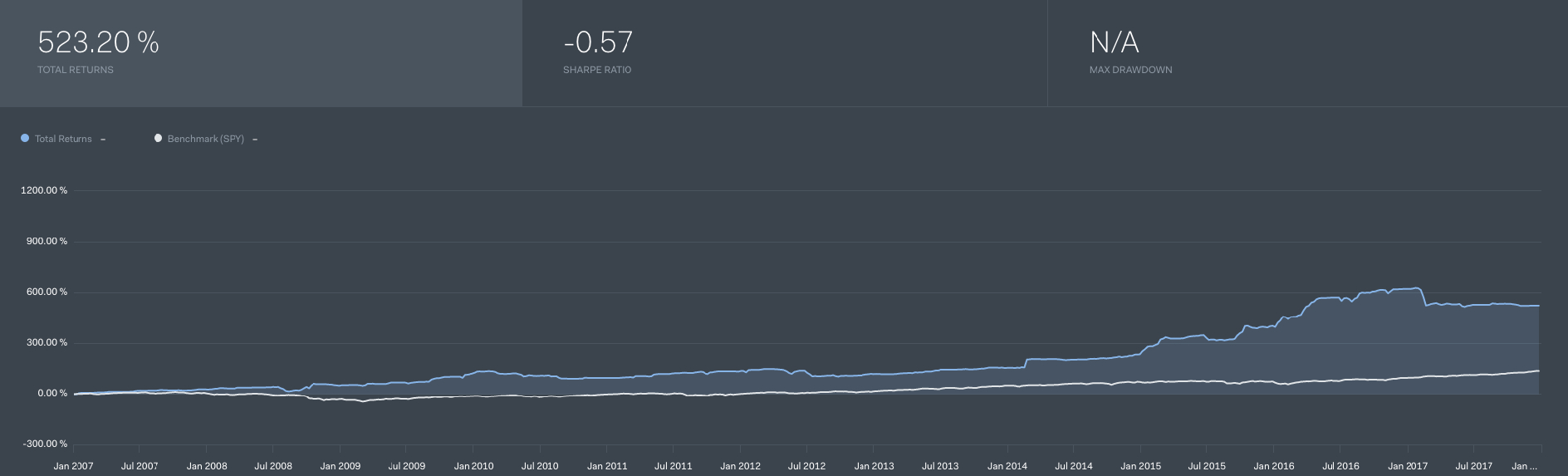Hi, I am trying to port this quantopian algo to quantconnect but not sure where to start. Quantopian does not do live trading for futures currently so I can only backtest this in quantopian

```#################################################################### # Futures momentum reversion trade algorithm # By Naoki Nagai, May 2017 ##################################################################### import numpy as np import scipy as sp from quantopian.algorithm import order_optimal_portfolio import quantopian.experimental.optimize as opt def initialize(context): # Futures to be traded by the algorithm, by asset class context.futures_by_assetclass = { 'equities' : [ 'SP', # S&P 500 Futures (US large cap) 'NK', # Nikkei 225 Futures (Japan) ], 'fixedincome': [ 'TU', # 2yr Tbill 'TY', # TNote 10 yr 'US', # TBond 30 yr 'ED', # Eurodollar ], 'currencies' : [ 'EC', # Euro 'JE', # Japanese YEN ], 'commodities' :[ 'CL', # Light Sweet Crude Oil 'GC', # Gold 'NG', # Natural gas 'CN', # Corn ], } # This holds all continuous future objects as an array context.futures = [] for assetclass in context.futures_by_assetclass: for future in context.futures_by_assetclass[assetclass]: context.futures.append(continuous_future(future)) # Window length for the trend. The algo checks the trend in this interval context.window = 63 # 63 days = one quarter # This arbitrary value determines the weight of futurues long and short context.multiplier = 2250. # Max leverage the algo can take context.maxleverage = 2.0 # How certain you want to be the trend is there. Null hypothesis probability context.pvalue = 0.15 # Rebalance every day, 30 minutes after market open schedule_function(func=rebalance, date_rule=date_rules.every_day(), time_rule=time_rules.market_open(minutes=30)) # Record exposure by asset class everyday schedule_function(record_exposure, date_rules.every_day(), time_rules.market_close()) def rebalance(context, data): # Calculate slopes for each futures prediction = calc_slopes(context, data) # Get target weights to futures contracts based on slopes target_weights = get_target_weights(context, data, prediction) # Exposure is noted for logging and record() plotting context.exposure = {} text = '' for contract in target_weights: context.exposure[contract.root_symbol] = target_weights[contract] if target_weights[contract] != 0: text += "\n%+3.1f%% \t%s \t(%s)" % (target_weights[contract]*100, contract.symbol, contract.asset_name) if text == '': text = '\nNo positions to take' log.info('Target position of today:' + text) # Rebalance portfolio using optimaize API order_optimal_portfolio( opt.TargetPortfolioWeights(target_weights), constraints=[opt.MaxGrossLeverage(context.maxleverage),], universe=target_weights ) def calc_slopes(context, data): # Initialize output prediction = {} # Get pricing data of continuous futures all_prices = data.history(context.futures, 'price', context.window + 1, '1d') # Calculate daily returns for each continuous futures all_returns = all_prices.pct_change()[1:] # for each future, run regression to underestand the trend of price movement for future in context.futures: # Y-axis is the daily return Y = np.array(all_returns[future]) # X-axis is -3, -2, -1, 0... X = np.array(range(-len(Y)+1,1)) # Then, we get a and b where Y = a X + b coef = sp.stats.linregress(X, Y) # Initialize prediction[future] = 0 # Return trend exists i.e. price momentum is accelerating with high probability if (coef.pvalue < context.pvalue): # Price momentumm is clear. Speed and acceleration is in same direction if (coef.slope * coef.intercept > 0.): # Then, predict the price trend should reverse prediction[future] = -coef.slope * context.multiplier return prediction def get_target_weights(context, data, prediction): # Target weights per contract target_weights = {} total = 0. for future in context.futures: total += prediction[future] # Target weight for the most traded actual futures contract for future in context.futures: # Get the contract from the continuous futures object contract = data.current(future, 'contract') # If contract is tradable, assign weight if contract and data.can_trade(contract): target_weights[contract] = prediction[future] / max(total,1.0) return target_weights def record_exposure(context, data): # Record net exposure to different asset classes for tracking for assetclass in context.futures_by_assetclass: # We add weights by asset class asset_weight = 0. for future in context.exposure: if future in context.futures_by_assetclass[assetclass]: asset_weight += context.exposure[future] # Plot exposure in asset class record(assetclass, asset_weight) # Record gross leverage record(leverage = context.account.leverage)```